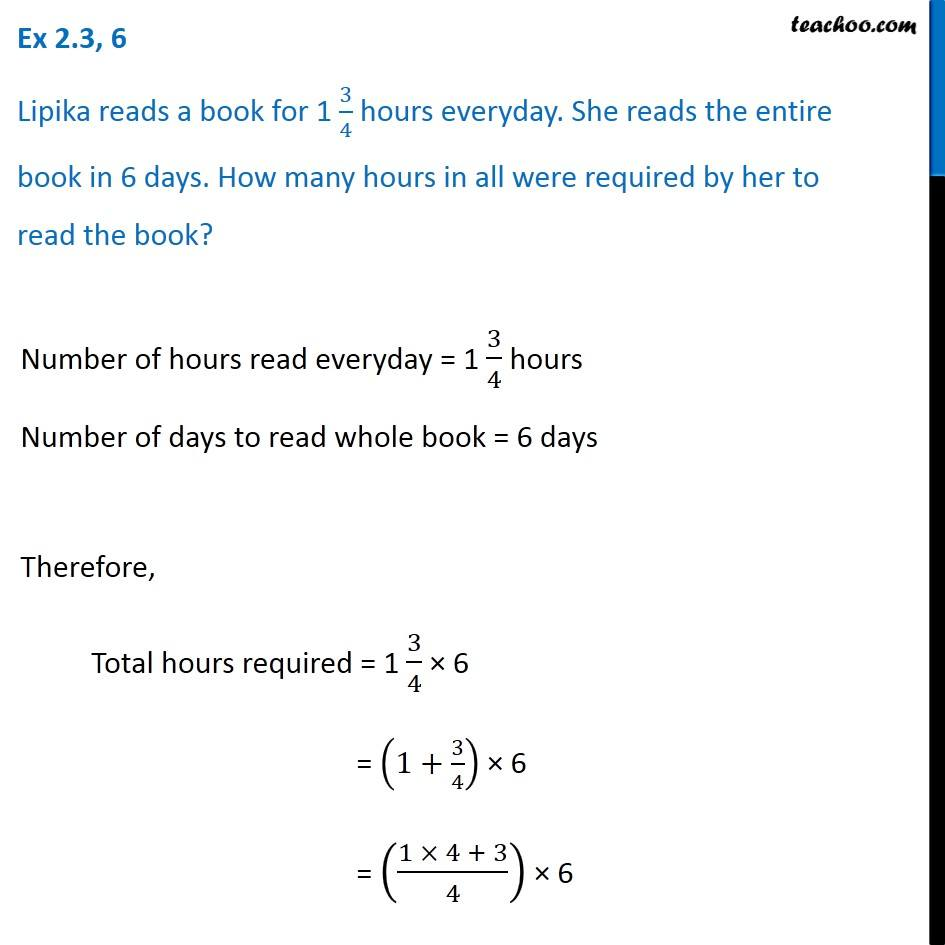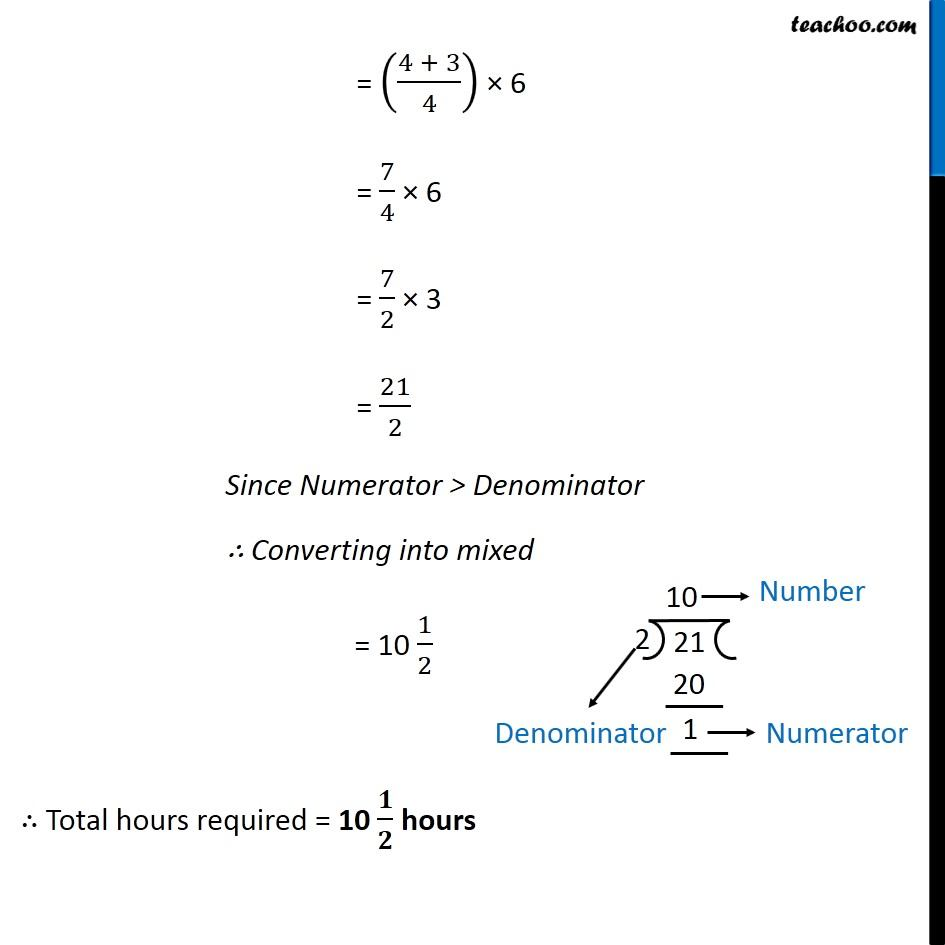Ex 2.3

Chapter 2 Class 7 Fractions and Decimals
Serial order wise641 students have Teachoo Black. What are you waiting for?

### Transcript

Ex 2.3, 6 Lipika reads a book for 1 3/4 hours everyday. She reads the entire book in 6 days. How many hours in all were required by her to read the book? Number of hours read everyday = 1 3/4 hours Number of days to read whole book = 6 days Therefore, Total hours required = 1 3/4 × 6 = (1+3/4) × 6 = ((1 × 4 + 3)/4) × 6 = ((4 + 3)/4) × 6 = 7/4 × 6 = 7/2 × 3 = 21/2 Since Numerator > Denominator ∴ Converting into mixed = 10 1/2 ∴ Total hours required = 10 𝟏/𝟐 hours